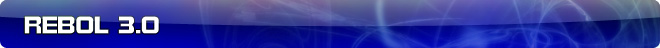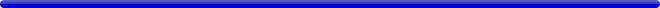# PARSE: CHECK vs IF, then EITHER

 Carl Sassenrath, CTO REBOL Technologies 22-Sep-2009 5:20 GMT Article #0247

The CHECK word has been proposed for parse:

```check (condition)
```

If the condition evaluates to true (using normal if rules), then parsing continues, otherwise the rule fails.

So, this expression parses an integer and checks that it is odd valued:

```[set i integer! check (odd? i)]
```

My initial reaction was that CHECK should be named IF, like the if function.

```[set i integer! if (odd? i)]
```

That seems good.

But, there's also a proposal for EITHER, which has the form:

```either rule1 rule2 rule3
```

It parses with rule1, and if true, then continues to parse with rule2, otherwise if rule1 is false, continues with rule3. This is not the same as:

```rule1 rule2 | rule3
```

nor:

```rule1 [rule2 | rule3]
```

but actually:

```rule1 rule2 | not rule1 rule3
```

But, EITHER is more efficient, because the NOT of rule1 is unnecessary.

However, in this case, the argument to EITHER is a rule block, not a paren expression as in IF above.

So, if we want IF, then is it problematic for EITHER not to work the same way (with an evaluated expression)? If so, then what do we want to call EITHER?